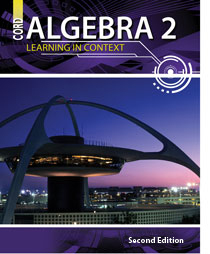# Algebra 2 - 2nd Edition

## Chapter 8: Systems of Equations and Inequalities

Some links are repeated for use with more than one lesson.

### The following links are applicable to the entire chapter.

http://www.coolmath.com/algebra/12-2x2-systems-of-equations/
This page contains an overview of the methods for solving systems of equations and inequalities, based on two equations and two unknowns.

http://www.explorelearning.com/index.cfm?CourseID=244&method=cResource.dspResourcesForCourse
This page contains several gizmos and activities for visualizing systems of equations and their solutions. This website requires Shockwave Player to run some of its features.

http://www.purplemath.com/modules/systlin1.htm
This series of pages contains a detailed tutorial on systems of equations. (Click Next)

http://www.regentsprep.org/Regents/math/ALGEBRA/AE3/indexAE3.htm
This series of pages has links to each method of solving systems of linear equations, as well as some interactive practice.

http://www.freemathhelp.com/Lessons/Algebra_1_Solving_Systems_of_Equations_BB.htm
This page has a video lecture and notes on solving systems of equations using one of three methods.

### 8.1 Solving Systems of Equations by Graphing

http://www.algebralab.org/studyaids/studyaid.aspx?file=Algebra1_7-1.xml
This page contains a tutorial on solving systems of linear equations by graphing.

http://mathbits.com/MathBits/TISection/Algebra1/systems.htm
This page describes how to use a graphing calculator to solve systems of equations.

### 8.2 Solving Systems of Equations Algebraically

http://www.sparknotes.com/math/algebra1/systemsofequations/section2.rhtml
This page demonstrates solving two systems of equations by the substitution method.

http://hotmath.com/help/gt/genericalg1/section_5_2.html
This page provides several practice problems for solving systems of equations by the substitution method.

### 8.3 Systems of Linear Inequalities

http://www.ronblond.com/M11/LinProg/
NEW This page contains an applet for graphing linear inequalities. This website requires Java to run some of its features.

http://www.explorelearning.com/index.cfm?method=cResource.dspDetail&ResourceID=90

### 8.4 Linear Programming

http://www.edhelper.com/LinearEquations.htm
This page contains links to free printable practice worksheets on linear programming, as well as other topics involving linear equations in this chapter.

http://teachers.henrico.k12.va.us/math/hcpsalgebra2/3-6.htm
This page contains resources for linear programming lessons including power point presentations and practice.

### 8.5 Solving Systems of Equations in Three Variables

http://www.mathwarehouse.com/algebra/planes/systems/three-variable-equations.php
This page contains 3D representations of different types of solutions and a tutorial on solving them.